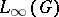The family of irreducible continuous unitary representations of a locally compact group, the non-zero matrix elements of which cannot be approximated by finite linear combinations of matrix elements of the regular representation ofin the topology of uniform convergence on compact sets in. The complementary series of the groupis non-empty if and only ifis not amenable, i.e. if the spacecontains no non-trivial left-invariant mean . A connected Lie grouphas a non-empty complementary series if and only if the semi-simple quotient group ofby its maximal connected solvable normal subgroup is non-compact (cf. Levi–Mal'tsev decomposition). A complementary series was first discovered for the complex classical groups . At the time of writing (1987) complementary series have been fully described only for certain locally compact groups. Certain problems in number theory (see, for example, ) are equivalent to problems in the theory of representations connected with the complementary series of adèle groups of linear algebraic groups.